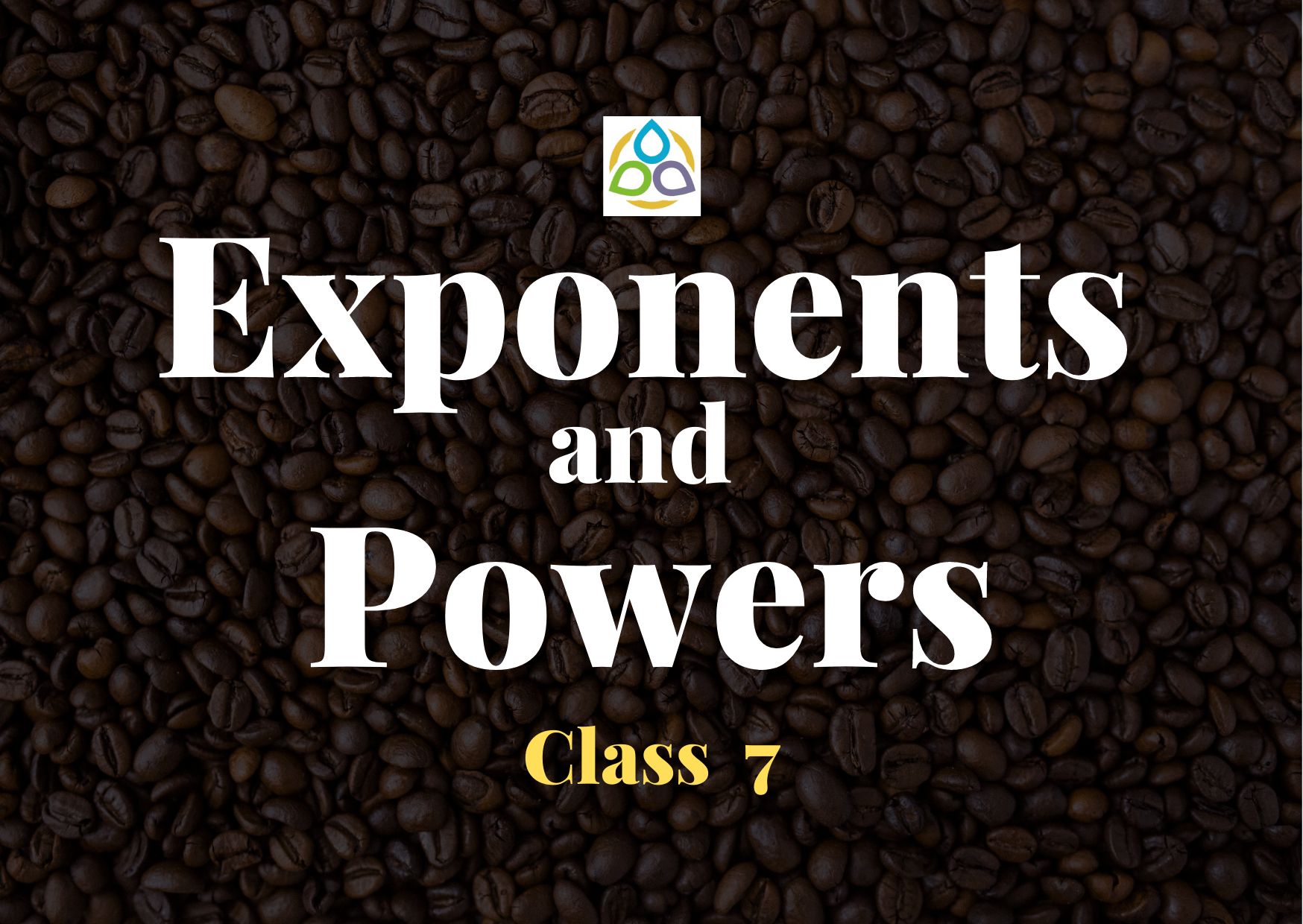# Exponents and Powers

15. If 5x = 53 × 56, the value of x is

• (A) 3
• (B) 0
• (C) 9
• (D) 10

14. If u = (–1)2022 and v = (–1)2023, then u + v is equal to

• (A) 0
• (B) 2
• (C) –1
• (D) 1

13. If u = (–1)2022 and v = (–1)2023, then u × v is equal to

• (A) –1
• (B) 2
• (C) 3
• (D) 1

12. If u = (–1)2022 and v = (–1)2023, then which among the following is true?

• (A) u = v
• (B) u > v
• (C) u < v
• (D) None of these

11. If 2n+2 – 2n+1 + 2n = c × 2n, find the value of c.

• (A) 0
• (B) 1
• (C) –1
• (D) 3

10. If 21998 – 21997 – 21996 + 21995 = K × 21995, then the value of K is

• (A) 1
• (B) 2
• (C) 3
• (D) 4

9. Value of (1022 + 1020)/1020 is

• (A) 10
• (B) 1042
• (C) 101
• (D) 1022

8. Value of (x12 + x10)/x10 is

• (A) x + 1
• (B) x2 + 1
• (C) x2
• (D) x12

7. Which of the following has the largest value?

• (A) 0.0001
• (B) 102/106
• (C) 1/106
• (D) (1/106) ÷ 0.1

6. Which of the following has the smallest value?

• (A) 0.001
• (B) 10/104
• (C) 1/105
• (D) (1/105) ÷ 0.1

5. Which among the following terms is largest?

• (A) (33)2
• (B) 35
• (C) 240
• (D) 23 × 33

4. Which among the following terms is smallest?

• (A) 22 × (22)3
• (B) 38 ÷ 33
• (C) 25+3
• (D) 25 × 32

3. By what number should we multiply 33 so that the product may be equal to 37?

• (A) 71
• (B) 81
• (C) 91
• (D) 82

2. By what number should (–4)5 be divided so that the quotient may be equal to (– 4)3?

• (A) (–4)2
• (B) (–4)5
• (C) (–4)3
• (D) (–4)0

1. How many times of 30 must be added together to get a sum equal to 307?

• (A) 30
• (B) 306
• (C) 303
• (D) 300